## ML Aggarwal Class 8 Solutions for ICSE Maths Chapter 10 Algebraic Expressions and Identities Check Your Progress

Question 1.
(i) -5x2y + 3xy2 – 7xy + 8, 12x2y – 5xy2 + 3xy – 2
(ii) 9xy + 3yz – 5zx, 4yz + 9zx – 5y, -5xz + 2x – 5xy
Solution: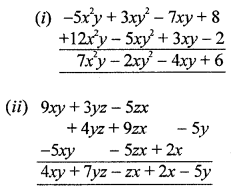Question 2.
Subtract:
(i) 5a + 3b + 11c – 2 from 3a + 5b – 9c + 3
(ii) 10x2 – 8y2 + 5y – 3 from 8x2 – 5xy + 2y+ 5x – 3y
Solution:
(i) 5a – 3b + 11c – 2 from 3a + 5b – 9c + 3(ii) 10x2 – 8y2 + 5y – 3 from 8x2 – 5xy + 2y2 + 5x – 3yQuestion 3.
What must be added to 5x2 – 3x + 1 to get 3x3 – 7x2 + 8?
Solution:
Required expression
= (3x3 – 7x2 + 8) – (5x2 – 3x + 1)
= 3x3 – 7x2 + 8 – 5x2 + 3x – 1
= 3x3 – 12x2 + 3x + 7

Question 4.
Find the product of
(i) 3x2y and -4xy2
(ii) –$$\frac{4}{5}$$xy, $$\frac{5}{7}$$yz and –$$\frac{14}{9}$$zx
Solution:
Product of
(i) 3x2y and -4xy2
= 3x2 × (-4xy2)
= -12x2+1 y1+2
= 12x3y3Question 5.
Multiply:
(i) (3pq – 4p2 + 5q2 + 7) by -7pq
(ii) ($$\frac{3}{4}$$x2y – $$\frac{4}{5}$$xy + $$\frac{5}{6}$$xy2) by – 15xyz
Solution:
(i) (3pq – 4p2 + 5q2 + 7) by -7pq
= -7pq × 3pq – 7pq × (-4p2) + (-7pq) (5q2) – 7pq × 7
= -21p2q2 + 28p3q – 35pq3 – 49pqQuestion 6.
Multiply:
(i) (5x2 + 4x – 2) by (3 – x – 4x2)
(ii) (7x2 + 12xy – 9y2) by (3x2 – 5xy + 3y2)
Solution:
(i) 5x2 + 4x – 2) by (3 – x – 4x2)
= 5x2(3 – x – 4x2) + 4x(3 – x – 4x2) – 2(3x – x – 4x2)
= 15x2 – 5x3 – 20x4 + 12x – 4x2 – 16x3 – 6x + 2x + 8x2
= -20x4 – 21x3 + 19x2 + 14x – 6

(ii) (7x2 + 12xy – 9y2) by (3x2 – 5xy + 3y2)
= 7x2(3x2 – 5xy + 3y2) + 12xy(3x2 – 5xy + 3y2) – 9y2(3x2 – 5xy + 3y2)
= 21x4 – 35x3y + 21x2y2 + 36x3y – 60x2y2 + 36xy3 – 27x2y2 + 45xy3 – 27y4
= 21x4 + x3y + 81xy3 – 66x2y2 – 27y4

Question 7.
Simplify the following expressions and evaluate them as directed:
(i) (3ab – 2a2 + 5b2) x (2b2 – 5ab + 3a2) + 8a3b – 7b4 for a = 1, b = -1
(ii) (1.7x – 2.5y) (2y + 3x + 4) – 7.8x2 – 10y for x = 0, y = 1.
Solution:
(i) (3ab – 2a2 + 5b2) × (2b2 – 5ab + 3a2) + 8a3b – 7b4
= 3ab(2b2 – 5ab + 3a2) – 2a2(2b2 – 5ab + 3a2) + 5b2(2b2 – 5ab + 3a2) + 8a3b – 7b4
= 6ab32 – 15a2b2 + 9a3b – 4a2b2 + 10a3b – 6a4 + 10b4 – 25ab3 + 15a2b2 + 8a3b – 7b4
= 27a3b – 4a2b2 – 19ab3 – 6a4 + 3b4
We have, a = 1, b = (-1)
= 27(1 )3 (-1) – 4(1)2 (-1)2 – 19 (1) (-1)3 – 6(1)4 + 3(-1)4
= -27 – 4 + 19 – 6 + 3
= -37 + 22 = -15

(ii) (1.7x – 2.5y) (2y + 3x + 4) – 7.8x2 – 10y
1.7x(2y + 3x + 4) – 2.5y(2y + 3x + 4) – 7.8x2 – 10y
= 3.4xy + 5.1x2 + 6.8x – 5y2 – 7.5xy – 10y – 7.8x2 – 10y
= -2.7x2 – 4.1xy – 5y2 + 6.8x – 20y
We have, x = 0, y = 1
= -2.7 × 0 – 4.1 × 0 × 1 – 5(1)2 + 6.8 × 0 – 20 × 1
= 0 + 0 – 5 + 0 – 20 = -25

Question 8.
Carry out the following divisions:
(i) 66pq2r3 ÷ 11qr2
(ii) (x3 + 2x2 + 3x) ÷ 2x
Solution:Question 9.
Divide 10x4 – 19x3 + 17x2 + 15x – 42 by 2x2 – 3x + 5.
Solution:
(10x4 – 19x3 + 17x2 + 15x – 42) ÷ (2x2 – 3x + 5)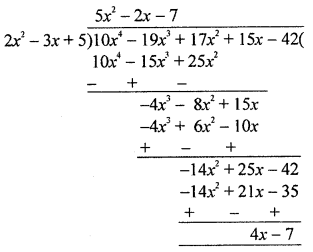Quotient = 5x2 – 2x – 7
Remainder = 4x – 7

Question 10.
Using identities, find the following products:
(i) (3x + 4y) (3x + 4y)
(ii) $$\left(\frac{5}{2} a-b\right)\left(\frac{5}{2} a-b\right)$$
(iii) (3.5m – 1.5n) (3.5m + 1.5n)
(iv) (7xy – 2)(7xy + 7)
Solution:
(i) (3x + 4y)(3x + 4y)
= (3x + 4y)2
= (3x)2 + 2 × 3x × 4y + (4y)2 {∵ (a + b)2 = a2 + 2ab + b2}
= 9x2 + 24xy + 16y2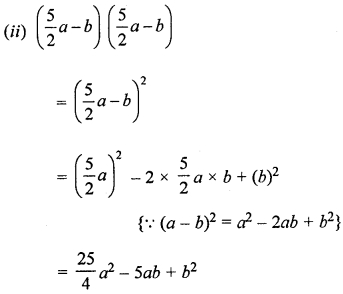(iii) (3.5m – 1.5n)(3.5m + 1.5n)
= (3.5m)2 – (1.5n)2 {∵ (a – b)(a + b) = a2 – b2}
= 12.25m2 – 2.25n2

(iv) (7xy – 2)(7xy + 7)
= (7xy)+ (-2 + 7) × (7xy) + (-2) × 7
{∵(x + a)(x + b) = x2 + (a + b)x + ab}
= 49x2y2 + 35xy – 14

Question 11.
Using suitable identities, evaluate the following:
(i) 1052
(ii) 972
(iii) 201 × 199
(iv) 872 – 132
(v) 105 × 107
Solution:
(i) (105)2 = (100 + 5)2
= (100)2 + 2 × 100 × 5 + (5)2
{(a + b)2 = a2 + 2ab + b2}
= 10000 + 1000 + 25 = 11025

(ii) (97)2 = (100 – 3)2
= (100)2 – 2 × 100 × 3 + (3)2
{(a – b)2 = a2 – 2ab + b2}
= 10000 – 600 + 9
= 10009 – 600 = 9409

(iii) 201 × 199 = (200 + 1) (200 – 1)
= (200)2 – (1)2
{(a + b) (a – b) = a2 – b2}
= 40000 – 1 = 39999

(iv) 872 – 132
= (87 + 13) (87- 13)
{a2 – b2 = (a + b) {a – b)}
= 100 × 74 = 7400

(v) 105 × 107 = (100 + 5) (100 + 7)
= (100)2 + (5 + 7) × 100 + 5 × 7
{(x + a)(x – b)
= x2 + (a + b)x + ab
= x2 + (a + b)x + ab}
= 10000 + 1200 + 35= 11235

Question 12.
Prove that following:
(i) (a + b)2 – (a – b)2 + 4ab
(ii) (2a + 3b)2 + (2a – 3b)2 = 8a2 + 18b2
Solution:
(i) (a + b)2 = (a – b)2 + 4ab
RHS = (a – b)2 + 4ab = a2– 2ab + b2 + 4ab
= a2 + 2ab + b2 = (a + b)2 = L.H.S.

(ii) (2a + 3b)2 + (2a – 3b)2 = 8a2 + 18b2
LHS = (2a + 3b)2 + (1a – 3b)2
= (2a)2 + 2 × 2a × 3b + (3b)2 + (2a)2 – 2 × 2a × 3b + (3b)2
= 4a2 + 12ab + 9b2 + 4a2 – 12ab + 9b2
= 8a2 + 18b2 = RHS

Question 13.
If x + $$\frac{1}{x}$$ = 5, evaluateSolution:
(i) x + $$\frac{1}{x}$$ = 5
Squaring both sides,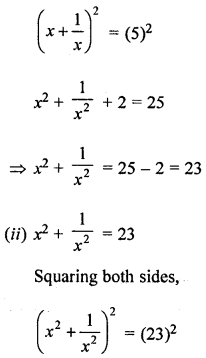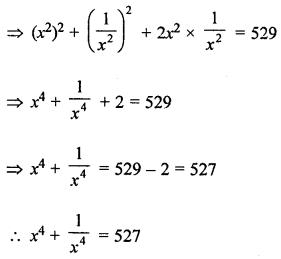Question 14.
If a + b = 5 and a2 + b2 = 13, find ab.
Solution:
a + b = 5 and a2 + b2 = 13
a + b = 5
Squaring both sides,
(a + b)2 = (5)2
a2 + b2 + 2ab = 25
13 + 2ab = 25 ⇒ 2ab = 25 – 13 = 12
⇒ ab = $$\frac{12}{2}$$ = 6
∴ ab = 6